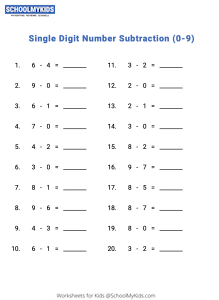# Math Worksheet : Single Digit Number Subtraction (0-9)

Single digit subtraction worksheets for Kindergarten and Grade 1 Students. Use these subtraction worksheets on single digit subtraction to teach kids subtract numbers less than 10. These printable single digit subtraction worksheets are part of our 1st Grade Math Subtraction Worksheets. This is the number 2 worksheet in a series of 5 worksheets on Subtraction within 10 (Single Digit Number Subtraction (0-9)).Viewed by 14448 parents and educators
Age Group
Subjects

#### Related Worksheets

Related worksheet for Kids

• Math